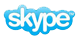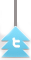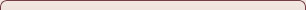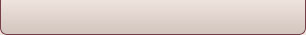# Question

1. Using appropriate diagrams, explain the difference between the law of diminishing returns and economies of scale. (nov 05)

1. Explain the relationship in the short-run between the marginal costs of a firm and its average total costs. (06?)

1. a) In the theory of the firm, a distinction is made between short-run cost curves and long-run cost curves. Using appropriate cost curve diagrams, explain this distinction.

b) Evaluate the view that greater economic efficiency will always be

achieved in perfect competition as compared to monopoly.

1.    a) Explain the differences between monopolistic competition and oligopoly as market structures.

b) Discuss the differences between a collusive and a non-collusive oligopoly.(Nov 05)

1. Using appropriate diagrams, explain whether a monopoly is likely to be more efficient or less efficient than a firm in perfect competition. (Paper 2, May 2006)